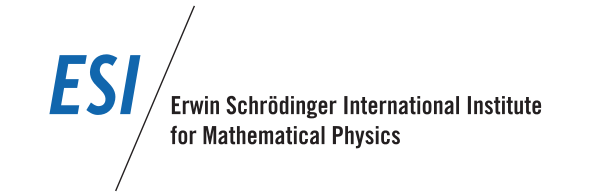# Workshop:Several complex variables and CR geometry

The topic of this workshop is the interaction between several complex variables and CR geometry and the connections to problems in mathematical physics. CR analysis refers to the study of the Cauchy-Riemann (or $\overline\partial$) equations on open subsets of $\mathbb C^n$, or to the equations they induce on real submanifolds of $\mathbb C^n$ (the tangential Cauchy-Riemann or $\overline\partial_b$ equations). This includes the study of functions in the kernels of these operators, that is, holomorphic functions and CR-functions respectively.

Our goal is to bring together researchers from complex analysis with expertise on the Cauchy-Riemann equations and their tangential variants (CR analysis) and experts in CR-geometry, in order to initiate a transfer of current ideas and methods between the two fields. CR analysis is also intimately related to diamagnetism, paramagnetism, and semiclassical analysis in quantum mechanics. We plan to use this workshop to rejuvenate and deepen the investigation of these connections.

In the first part (Nov. 03 - Nov 06, 2015) the focus will be on more geometric aspects, in the second part (Nov. 09 - Nov.13, 2015) on more analytic aspects.

## The basics

 When: Nov. 03, 2015 to Nov. 13, 2015 Where: ESI, Boltzmann Lecture Hall Organizers: Siqi Fu (Rutgers U, Camden), Friedrich Haslinger (U Vienna), Bernard Lamel (U Vienna), Emil Straube (Texas A & M U) LocalOrganizers: Franz Berger (U Vienna), Ilya Kossovskiy (U Vienna)